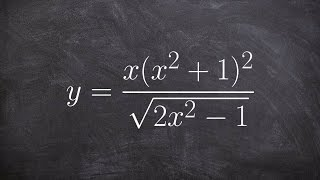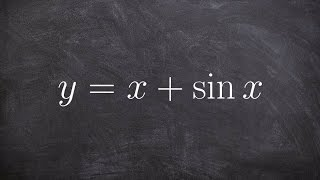# Related Videos

## Find the derivative of $\arctan\left(\frac{x}{y}\right)$

Go!
1
2
3
4
5
6
7
8
9
0
a
b
c
d
f
g
m
n
u
v
w
x
y
z
.
(◻)
+
-
×
◻/◻
/
÷
2

e
π
ln
log
log
lim
d/dx
Dx
|◻|
=
>
<
>=
<=
sin
cos
tan
cot
sec
csc

asin
acos
atan
acot
asec
acsc

sinh
cosh
tanh
coth
sech
csch

asinh
acosh
atanh
acoth
asech
acsch

### Videos### Calculus - Take the log of both sides to find the derivative, y = (x(x^2 + 1)^2)/(sqrt(2x^2 - 1))### Calculus: Derivatives 2 | Taking derivatives | Differential Calculus | Khan Academy### Calculus - Find the point where their exist a horizontal tangent line, y = x + sinx### Implicit differentiation | Advanced derivatives | AP Calculus AB | Khan Academy### Worked example: Evaluating derivative with implicit differentiation | AP Calculus AB | Khan Academy### Second derivatives (implicit equations): find expression | AP Calculus AB | Khan Academy

$\frac{d}{dx}\left(arctan\left(\frac{x}{y}\right)\right)$